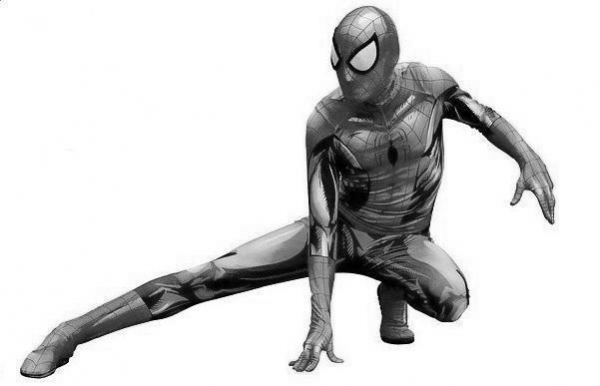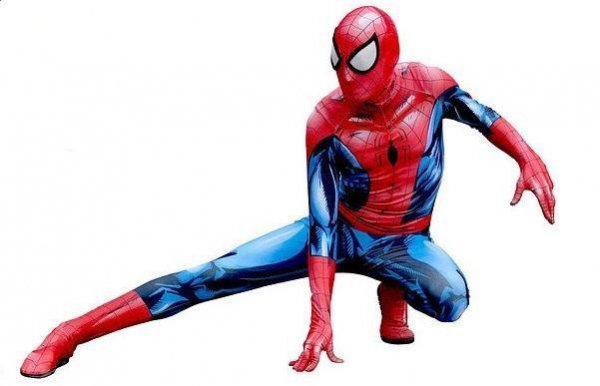# How to compare two images using Java OpenCV library?

To compare two images −

• Get the height and width of both of them to make sure they are equal.

• Get the pixel values and, get the RGB values of both of the images.

• Get the sum of the differences between the RGB values of these two images.

• Calculate the percentage of the difference using the following formula −

Average = difference/weight*height*3;
Percentage = (Average/255)*100;

## Example

import java.awt.Color;
import java.awt.image.BufferedImage;
import javax.imageio.ImageIO;
import java.io.File;
public class ComparingImages {
public static void main(String[] args) throws Exception {
int w1 = img1.getWidth();
int w2 = img2.getWidth();
int h1 = img1.getHeight();
int h2 = img2.getHeight();
if ((w1!=w2)||(h1!=h2)) {
System.out.println("Both images should have same dimwnsions");
} else {
long diff = 0;
for (int j = 0; j < h1; j++) {
for (int i = 0; i < w1; i++) {
//Getting the RGB values of a pixel
int pixel1 = img1.getRGB(i, j);
Color color1 = new Color(pixel1, true);
int r1 = color1.getRed();
int g1 = color1.getGreen();
int b1 = color1.getBlue();
int pixel2 = img2.getRGB(i, j);
Color color2 = new Color(pixel2, true);
int r2 = color2.getRed();
int g2 = color2.getGreen();
int b2= color2.getBlue();
//sum of differences of RGB values of the two images
long data = Math.abs(r1-r2)+Math.abs(g1-g2)+ Math.abs(b1-b2);
diff = diff+data;
}
}
double avg = diff/(w1*h1*3);
double percentage = (avg/255)*100;
System.out.println("Difference: "+percentage);
}
}
}

## Input1## Input2## Output

Difference: 92.54901960784314

Updated on: 09-Apr-2020

4K+ Views